##Trilinear polar and homographies

There is an aspect of the trilinear polar tr(K) of a point K with respect to a triangle ABC connecting it with homographies. In fact, the system (ABC,K) of a triangle and a point uniquely defines a homography F (perspectivity) fixing K and mapping {A,B,C} to the traces {A',B',C'} of K.
This homography is conjugate F=G*F0*G-1 to the antihomothety F0 of the equilateral to its medial triangle. G is the projectivity mapping the system (A0B0C0,O) of the equilateral triangle and its center to (ABC, K). It follows immediately that F is a homology (see Perspectivity.html ) with coefficient k=-2 having one isolated fixed point K and a line consisting entirely of fixed points which is no other than the trilinear polar tr(K) with respect to ABC.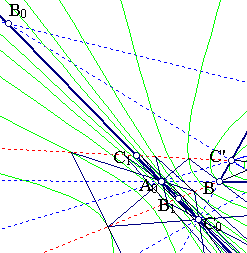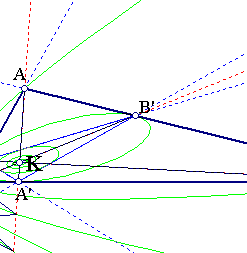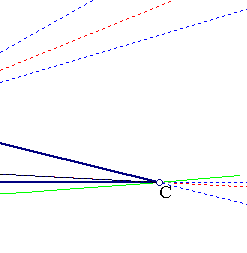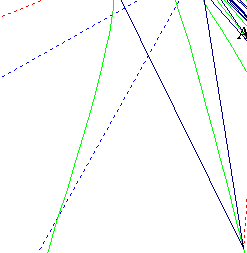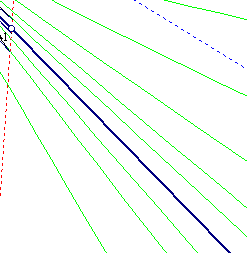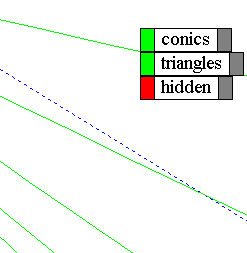The figure shows part of the sequence of triangles resulting by applying F and F-1 repeatedly to ABC. All resulting triangles have their vertices on the lines {KA,KB,KC}. The sides of all these triangles pass through three points {A0,B0,C0} on the trilinear polar tr(K).
Applying repeatedly F and F-1 to the circumconic c0 of ABC with perspector K (see TriangleConics.html ) we obtain also a sequence of conics in which is also contained the dual c1 of c0. These two sequences and their properties are discussed in some extend in IsotomicGeneral.html .